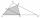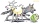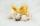# MO - triangles

On the AB and AC sides of the triangle ABC lies successive points E and F, on segment EF lie point D. The EF and BC lines are parallel and is true this ratio FD:DE = AE:EB = 2:1. The area of ABC triangle is 27 hectares and line segments EF, AD, and DB segments are divided into four parts. Find the areas of these four parts.

Result

AED =  4 ha
BDE =  2 ha
BCFD =  13 ha

#### Solution:Leave us a comment of example and its solution (i.e. if it is still somewhat unclear...):Be the first to comment!## Next similar examples:

1. Rectangular trianglesThe lengths of corresponding sides of two rectangular triangles are in the ratio 2:5. At what ratio are medians relevant to hypotenuse these right triangles? At what ratio are the contents of these triangles? Smaller rectangular triangle has legs 6 and 8 cThe shadow of the tree is 16 meters long. Shadow of two meters high tourist sign beside standing is 3.2 meters long. What height has tree (in meters)?
3. Isosceles trapezoidIn an isosceles trapezoid KLMN intersection of the diagonals is marked by the letter S. Calculate the area of trapezoid if /KS/: /SM/ = 2:1 and a triangle KSN is 14 cm2.
4. The farmerThe farmer would like to first seed his small field. The required amount depends on the seed area. Field has a triangular shape. The farmer had fenced field, so he knows the lengths of the sides: 119, 111 and 90 meters. Find a suitable way to determine th
5. Katy MOKate draw triangle ABC. Middle of AB have mark as X and the center of the side AC as Y. On the side BC wants to find the point Z such that the content area of a 4gon AXZY was greatest. What part of the triangle ABC can maximally occupy 4-gon AXZY?
6. MedianThe median of the triangle LMN is away from vertex N 84 cm. Calculate the length of the median, which start at N.
7. Center traverseIt is true that the middle traverse bisects the triangle?
8. An equilateralAn equilateral triangle with a side 10 m represents a wooden platform standing in a lawn. A goat is tied to a corner with a 15 m rope. What is the maximum amount of grazing area available to the goat?
9. SinesIn ▵ ABC, if sin(α)=0.5 and sin(β)=0.6 calculate sin(γ)
10. AceThe length of segment AB is 24 cm and the point M and N divided it into thirds. Calculate the circumference and area of this shape.
11. Theorem proveWe want to prove the sentence: If the natural number n is divisible by six, then n is divisible by three. From what assumption we started?
12. Roof tilesThe roof has a trapezoidal shape with bases of 15 m and 10 m, height of roof is 4 meters. How many tiles will need if on 1 m2 should be used 8 tiles?
13. TunnelsMice had built an underground house consisting of chambers and tunnels: • each tunnel leading from the chamber to the chamber (none is blind) • from each chamber lead just three tunnels into three distinct chambers, • from each chamber mice can get to any
14. 6 termsFind the first six terms of the sequence. a1 = 7, an = an-1 + 6
15. PowersExpress the expression ? as the n-th power of the base 10.
16. First manWhat is the likelihood of a random event where are five men and seven women first will leave the man?
17. One halfOne half of ? is: ?